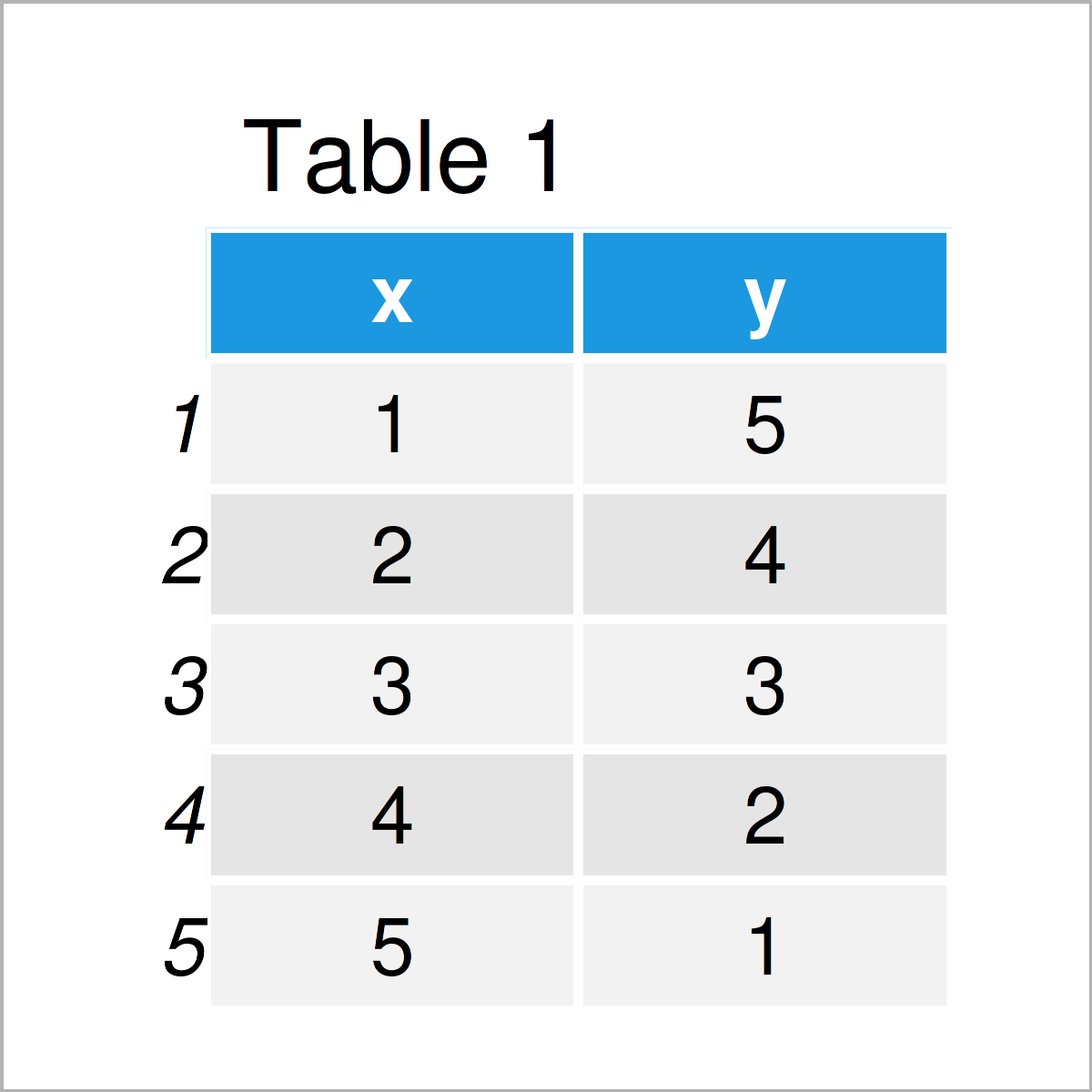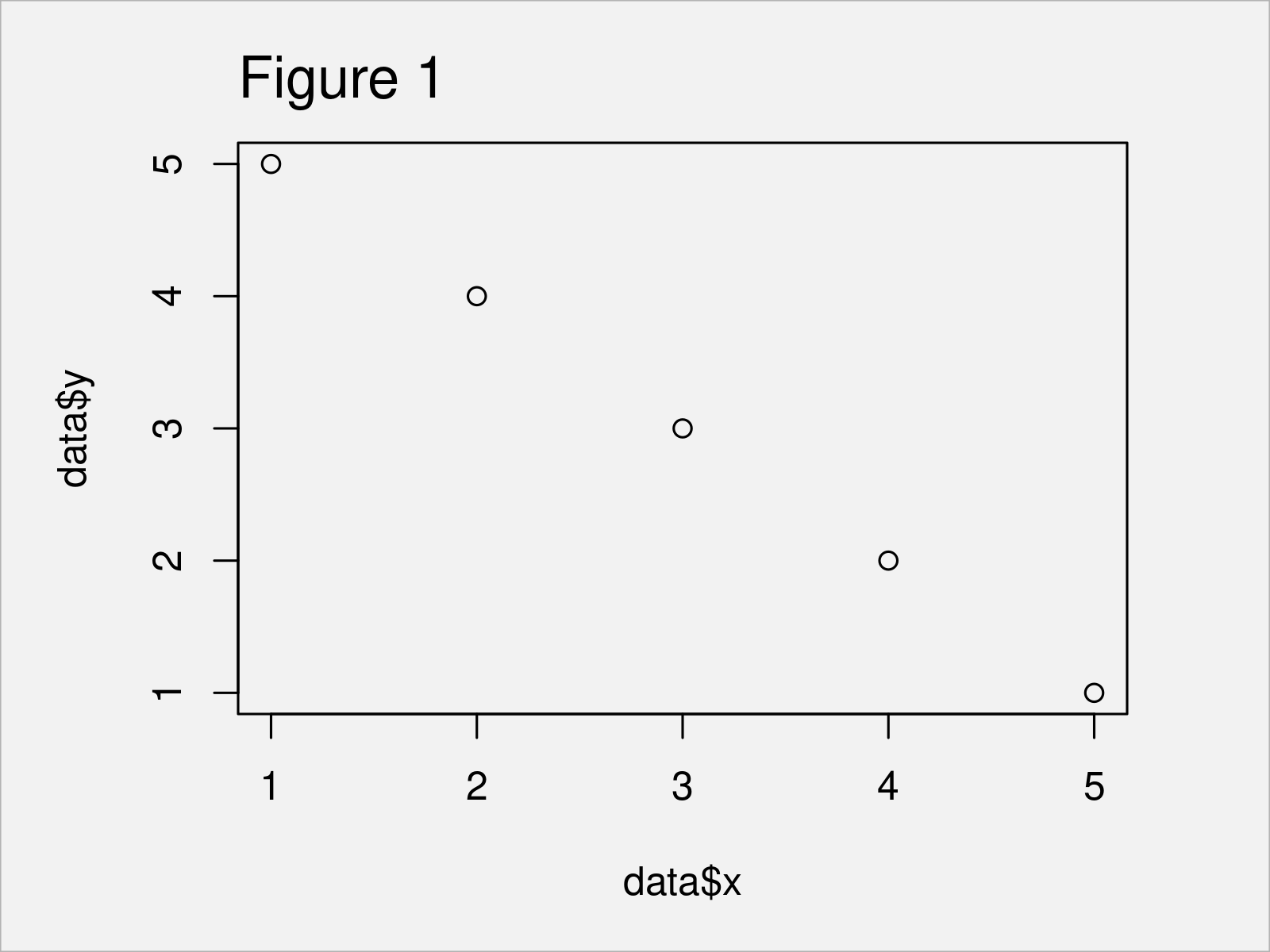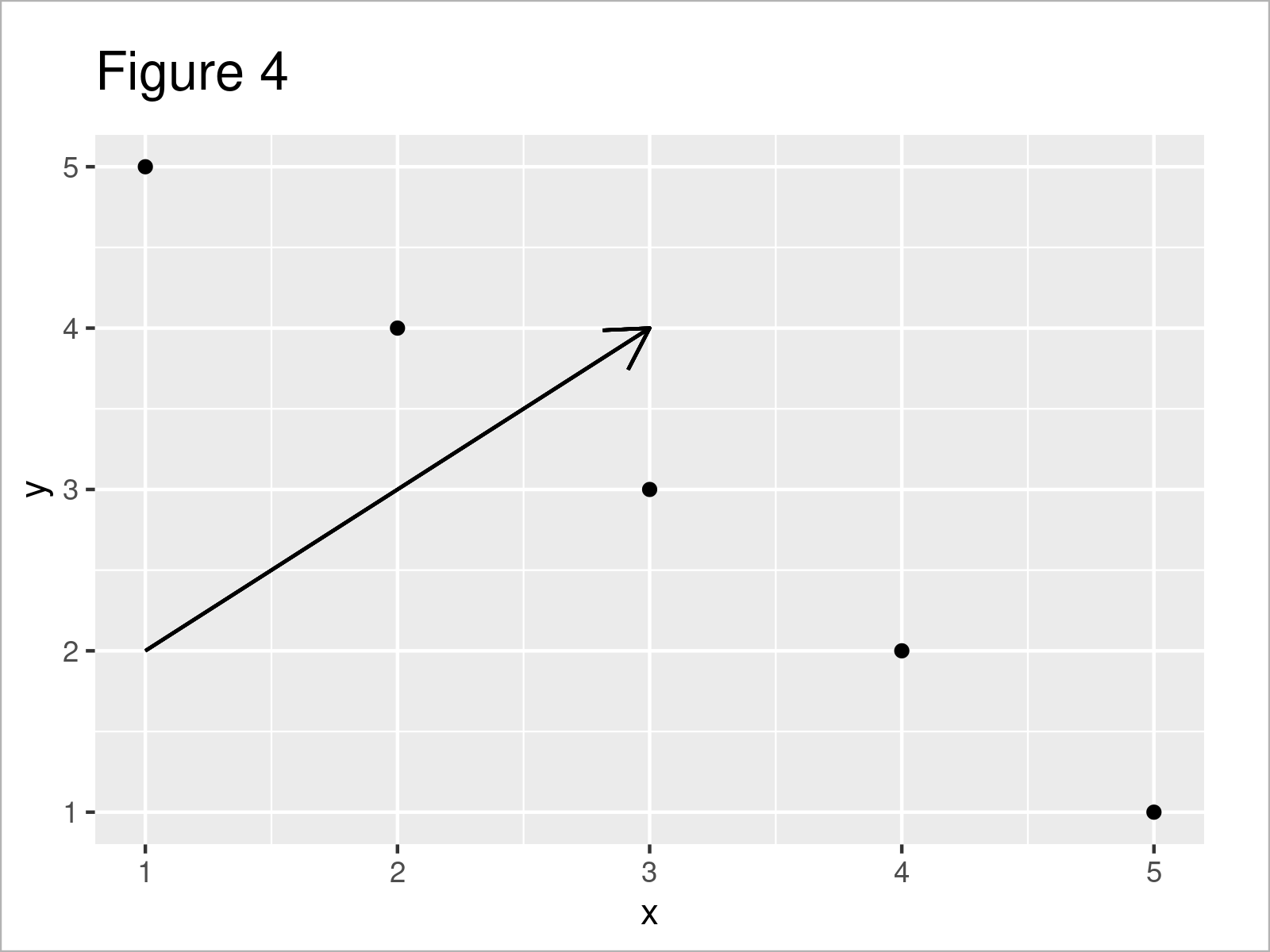# Add Arrow to Plot in R (2 Examples)

This article shows how to draw a graphic with an arrow in the R programming language.

The tutorial consists of these contents:

Let’s dive into it:

## Example Data

The first step is to create some example data:

```data <- data.frame(x = 1:5,         # Construct example data
y = 5:1)
data                                # Print example data```As you can see based on Table 1, our example data is a data frame comprising five rows and two integer columns with the names “x” and “y”.

## Example 1: Draw Base R Plot with Arrow Using arrows() Function

Example 1 illustrates how to add an arrow to a Base R plot.

Consider the following example plot:

```plot(data\$x,                        # Draw Base R plot without arrow
data\$y)```As shown in Figure 1, we have created a scatterplot without an arrow with the previous R syntax.

If we would like to add an arrow to this plot, we could apply the arrow function as shown below:

```plot(data\$x,                        # Draw Base R plot with arrow
data\$y)
arrows(x0 = 1,
y0 = 2,
x1 = 3,
y1 = 4)```In Figure 2 it is shown that we have created a scatterplot with arrow by running the previous R programming code.

## Example 2: Draw ggplot2 Plot with Arrow Using geom_segment() Function

In this section, I’ll illustrate how to draw a ggplot2 plot with an arrow.

First, we have to install and load the ggplot2 package:

```install.packages("ggplot2")         # Install ggplot2 package

Next, we can draw a ggplot2 scatterplot without arrow as shown below:

```ggp <- ggplot(data, aes(x, y)) +    # Draw ggplot2 plot without arrow
geom_point()
ggp```Note that the previous R code has created a plot object called ggp.

In the next step, we can use this plot object as basement for the geom_segment function. Within this function, we can specify the coordinates of the arrow that we want to create:

```ggp +                               # Draw ggplot2 plot with arrow
geom_segment(aes(x = 1,
y = 2,
xend = 3,
yend = 4),
arrow = arrow(length = unit(0.5, "cm")))```As shown in Figure 4, the previous R syntax has drawn a ggplot2 plot with an arrow.

## Video & Further Resources

If you need further instructions on the R programming codes of this article, I recommend watching the following video on my YouTube channel. In the video, I’m explaining the content of this tutorial:

In addition, you may want to have a look at some of the other tutorials on this website.

In this R tutorial you have learned how to add a nice and beautiful arrow to a graph.

Please note that it would be possible to modify the attributes of these arrows such as the shape, size, color, and line type. Have a look at the corresponding help documentations for more info.